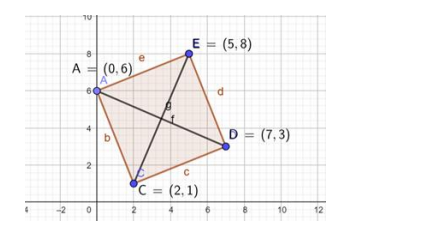Deepak Scored 45->99%ile with Bounce Back Crack Course. You can do it too!

# Show that the points A(0, 6), B(2, 1) and C(7, 3) are three corners

Question:

Show that the points A(0, 6), B(2, 1) and C(7, 3) are three corners of a square ABCD. Find (i) the slope of the diagonal BD and (ii) the coordinates of the fourth vertex D.

Solution:

In a square, all sides are perpendicular to the adjacent side, so the product of slope of two adjacent sides is -1.

Let the position of point $D(a, b)$.

Given points of the square are $\mathrm{A}(0,6), \mathrm{B}(2,1), \mathrm{C}(7,3)$ and $\mathrm{D}(\mathrm{a}, \mathrm{b})$.

slope $=\left(\frac{\mathrm{y}_{2}-\mathrm{y}_{1}}{\mathrm{x}_{2}-\mathrm{x}_{1}}\right)$The slope of line $A B=\left(\frac{1-6}{2-0}\right)=\frac{-5}{2}=m_{1}$

The slope of line $B C=\left(\frac{3-1}{7-2}\right)=\frac{2}{5}=m_{2}$

The slope of line $C D=\left(\frac{b-3}{a-7}\right)=m_{3}$

The slope of line $D A=\left(\frac{b-6}{a-0}\right)=\frac{b-6}{a}=m_{4}$

The slope of diagonal $A C=\left(\frac{3-6}{7-0}\right)=\frac{-3}{7}$

The slope of diagonal $\mathrm{BD}=\mathrm{m}_{5}$

(i) We know that in a square, two diagonals are perpendicular to each other, therefore

The slope of diagonal $A C \times$ slope of diagonal $B D=-1$

$\mathrm{m}_{5} \times \frac{-3}{7}=-1$

$\Rightarrow \mathrm{m}_{5}=\frac{7}{3}$

So the slope of diagonal BD is 7/3.

(ii) We know that midpoint of diagonal $A C=$ midpoint of diagonal $B D$

$0\left(\frac{x_{1}+x_{2}}{2}, \frac{y_{1}+y_{2}}{2}\right)$ and comparing $x$ and $y$ coordinates respectively.

$\left(\frac{7+0}{2}, \frac{3+6}{2}\right)=\left(\frac{a+2}{2}, \frac{b+1}{2}\right)$

$\Rightarrow\left(\frac{7}{2}, \frac{9}{2}\right)=\left(\frac{a+2}{2}, \frac{b+1}{2}\right)$

$\Rightarrow \frac{7}{2}=\frac{a+2}{2} \& \frac{9}{2}=\frac{b+1}{2}$

$\Rightarrow a=5 \& b=8$

So coordinate of the point D(5,8)